# Basics of Quantum Mechanics Basics of Quantum Mechanics

• Slides: 36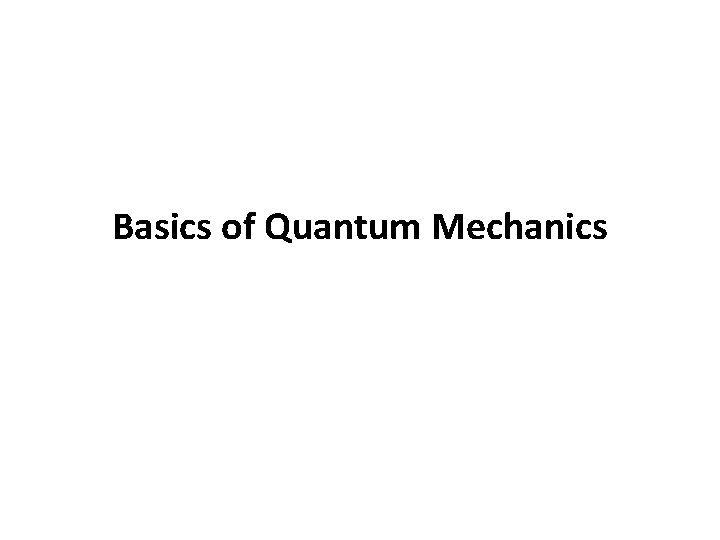Basics of Quantum Mechanics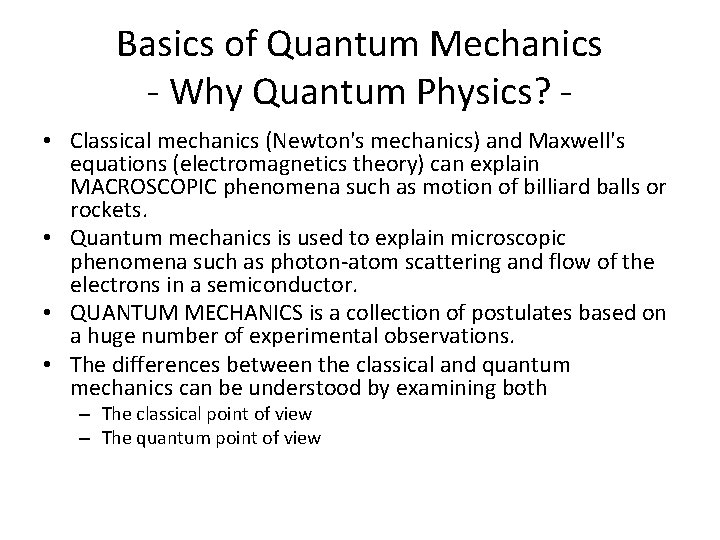Basics of Quantum Mechanics - Why Quantum Physics? • Classical mechanics (Newton's mechanics) and Maxwell's equations (electromagnetics theory) can explain MACROSCOPIC phenomena such as motion of billiard balls or rockets. • Quantum mechanics is used to explain microscopic phenomena such as photon-atom scattering and flow of the electrons in a semiconductor. • QUANTUM MECHANICS is a collection of postulates based on a huge number of experimental observations. • The differences between the classical and quantum mechanics can be understood by examining both – The classical point of view – The quantum point of view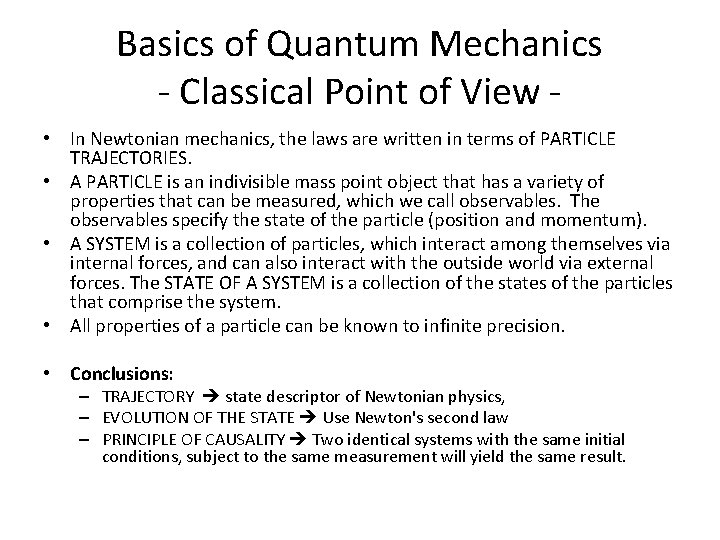Basics of Quantum Mechanics - Classical Point of View • In Newtonian mechanics, the laws are written in terms of PARTICLE TRAJECTORIES. • A PARTICLE is an indivisible mass point object that has a variety of properties that can be measured, which we call observables. The observables specify the state of the particle (position and momentum). • A SYSTEM is a collection of particles, which interact among themselves via internal forces, and can also interact with the outside world via external forces. The STATE OF A SYSTEM is a collection of the states of the particles that comprise the system. • All properties of a particle can be known to infinite precision. • Conclusions: – TRAJECTORY state descriptor of Newtonian physics, – EVOLUTION OF THE STATE Use Newton's second law – PRINCIPLE OF CAUSALITY Two identical systems with the same initial conditions, subject to the same measurement will yield the same result.Basics of Quantum Mechanics - Quantum Point of View • Quantum particles can act as both particles and waves WAVE-PARTICLE DUALITY • Quantum state is a conglomeration of several possible outcomes of measurement of physical properties Quantum mechanics uses the language of PROBABILITY theory (random chance) • An observer cannot observe a microscopic system without altering some of its properties. Neither one can predict how the state of the system will change. • QUANTIZATION of energy is yet another property of "microscopic" particles.Basics of Quantum Mechanics - Heisenberg Uncertainty Principle • One cannot unambiguously specify the values of particle's position and its momentum for a microscopic particle, i. e. • Position and momentum are, therefore, considered as incompatible variables. • The Heisenberg uncertainty principle strikes at the very heart of the classical physics => the particle trajectory.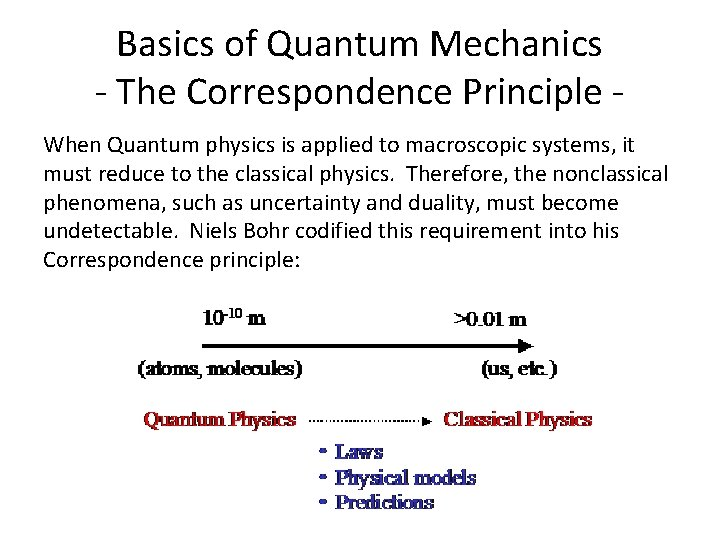Basics of Quantum Mechanics - The Correspondence Principle When Quantum physics is applied to macroscopic systems, it must reduce to the classical physics. Therefore, the nonclassical phenomena, such as uncertainty and duality, must become undetectable. Niels Bohr codified this requirement into his Correspondence principle:Basics of Quantum Mechanics - Particle-Wave Duality • The behavior of a "microscopic" particle is very different from that of a classical particle: – in some experiments it resembles the behavior of a classical wave (not localized in space) – in other experiments it behaves as a classical particle (localized in space) • Corpuscular theories of light treat light as though it were composed of particles, but can not explain DIFRACTION and INTERFERENCE. • Maxwell's theory of electromagnetic radiation can explain these two phenomena, which was the reason why the corpuscular theory of light was abandoned.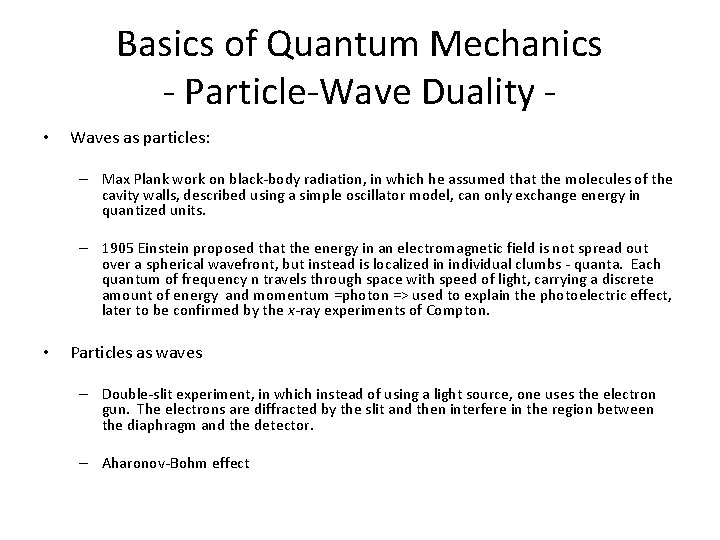Basics of Quantum Mechanics - Particle-Wave Duality • Waves as particles: – Max Plank work on black-body radiation, in which he assumed that the molecules of the cavity walls, described using a simple oscillator model, can only exchange energy in quantized units. – 1905 Einstein proposed that the energy in an electromagnetic field is not spread out over a spherical wavefront, but instead is localized in individual clumbs - quanta. Each quantum of frequency n travels through space with speed of light, carrying a discrete amount of energy and momentum =photon => used to explain the photoelectric effect, later to be confirmed by the x-ray experiments of Compton. • Particles as waves – Double-slit experiment, in which instead of using a light source, one uses the electron gun. The electrons are diffracted by the slit and then interfere in the region between the diaphragm and the detector. – Aharonov-Bohm effect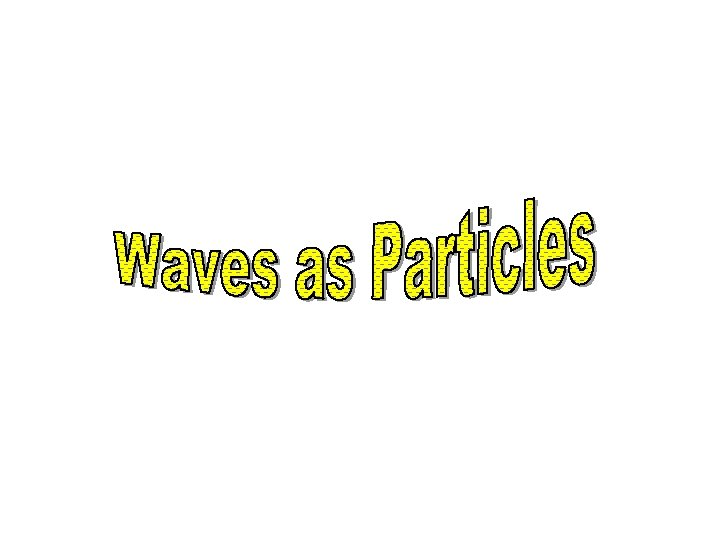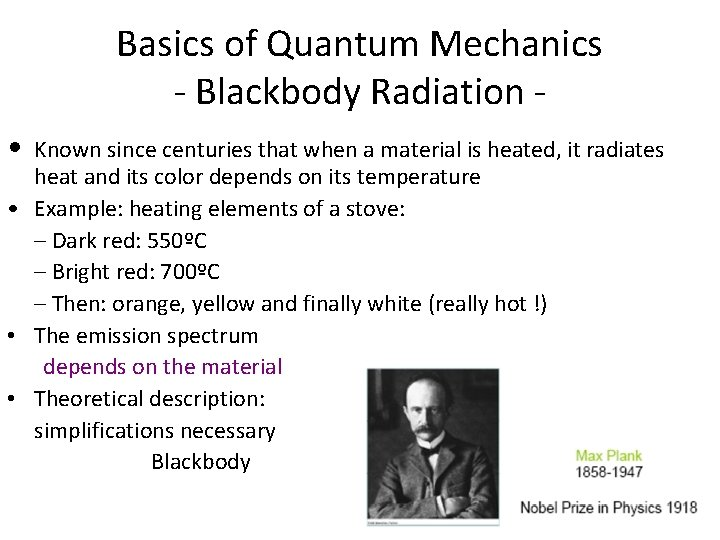Basics of Quantum Mechanics - Blackbody Radiation • Known since centuries that when a material is heated, it radiates heat and its color depends on its temperature • Example: heating elements of a stove: – Dark red: 550ºC – Bright red: 700ºC – Then: orange, yellow and finally white (really hot !) • The emission spectrum depends on the material • Theoretical description: simplifications necessary BlackbodyBlackbody? • A material is constantly exchanging heat with its surrounding (to remain at a constant temperature): – It absorbs and emits radiations – Problem: it can reflect incoming radiations, which makes a theoretical description more difficult (depends on the environment) • A blackbody is a perfect absorber: – Incoming radiations is totally absorbed and none is reflectedBlackbody Radiation • Blackbody = a cavity, such as a metal box with a small hole drilled into it. – Incoming radiations entering the hole keep bouncing around inside the box with a negligible change of escaping again through the hole => Absorbed. – The hole is the perfect absorber, e. g. the blackbody Radiation emission does not depend on the material the box is made of => Universal in nature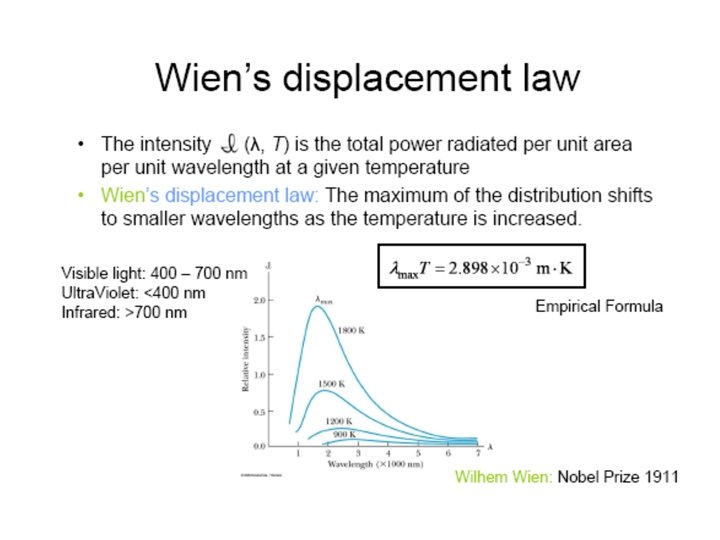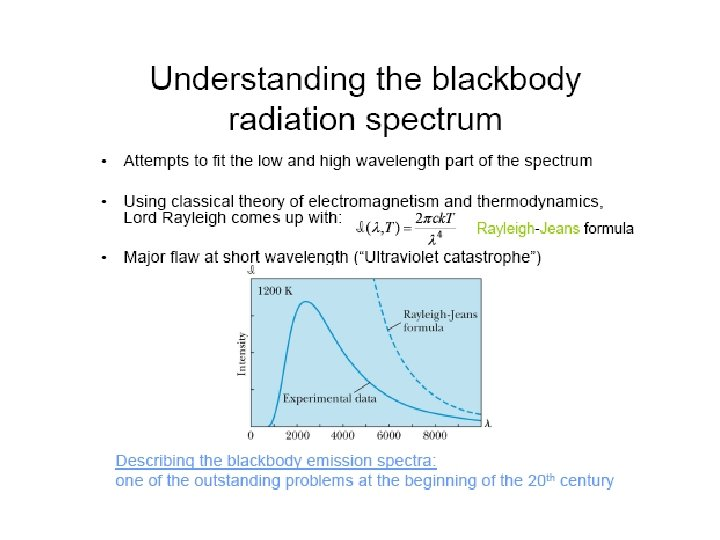Two Catastrophes? • Classical physics: – Emission spectrum: a superposition of electromagnetic waves of different frequencies – Frequencies allowed: standing waves inside the cavity • Equipartition of the energy: – Every standing wave carries k. T of energy – Flaw: when l → 0, the number of standing waves ↑, leading to E → ∞ • [Ultraviolet Catastrophe] Failure of classical theories: – The work of Rayleigh-Jeans was considered as state-of-the-art, using well tested theories, which were in very good agreement with experimental results in many other circumstances. – Need for a new theory…Basics of Quantum Mechanics - Photoelectric Effect A Photocell is Used to Study the Photoelectric Effect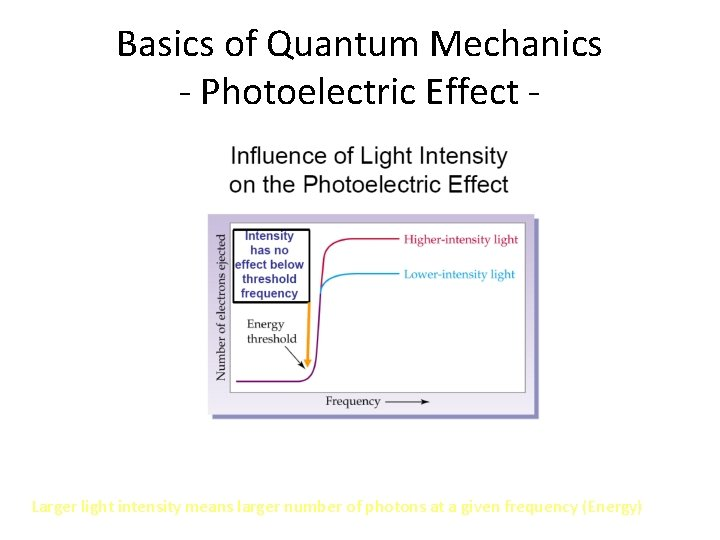Basics of Quantum Mechanics - Photoelectric Effect - Larger light intensity means larger number of photons at a given frequency (Energy)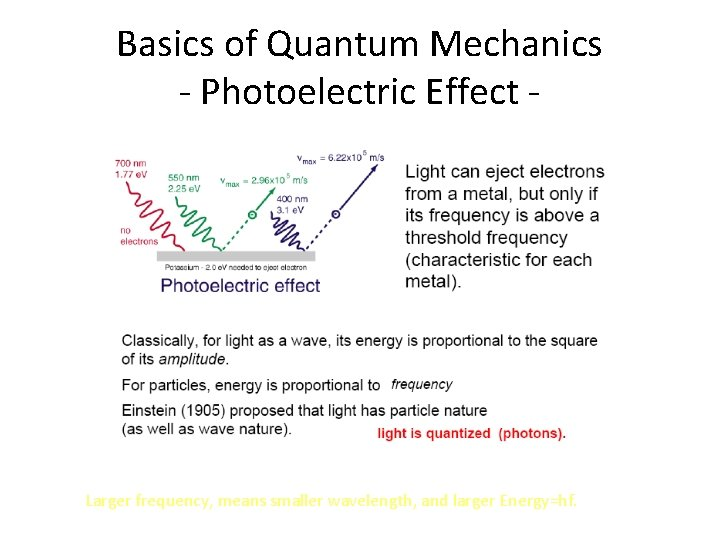Basics of Quantum Mechanics - Photoelectric Effect - Larger frequency, means smaller wavelength, and larger Energy=hf.Basics of Quantum Mechanics - Photoelectric Effect – – – The photoelectric effect provides evidence for the particle nature of light. It also provides evidence for quantization. If light shines on the surface of a metal, there is a point at which electrons are ejected from the metal. The electrons will only be ejected once threshold frequency is reached. Below the threshold frequency, no electrons are ejected. Above threshold frequency, the number of electrons ejected depend on the intensity of the light.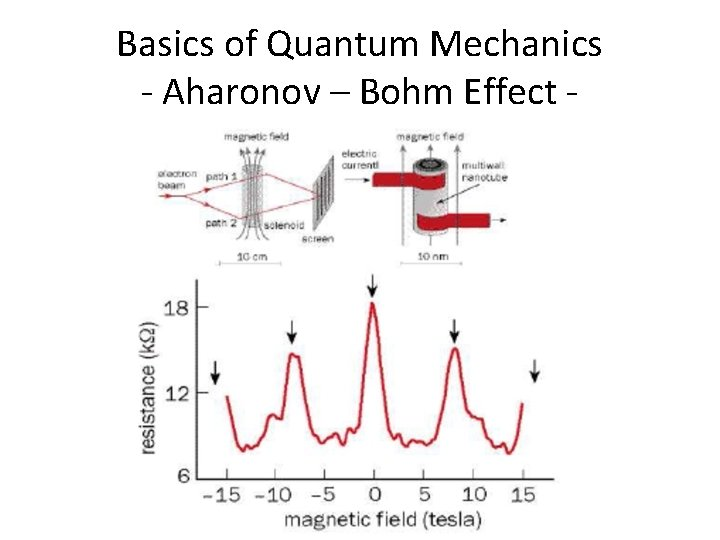Basics of Quantum Mechanics - Aharonov – Bohm Effect -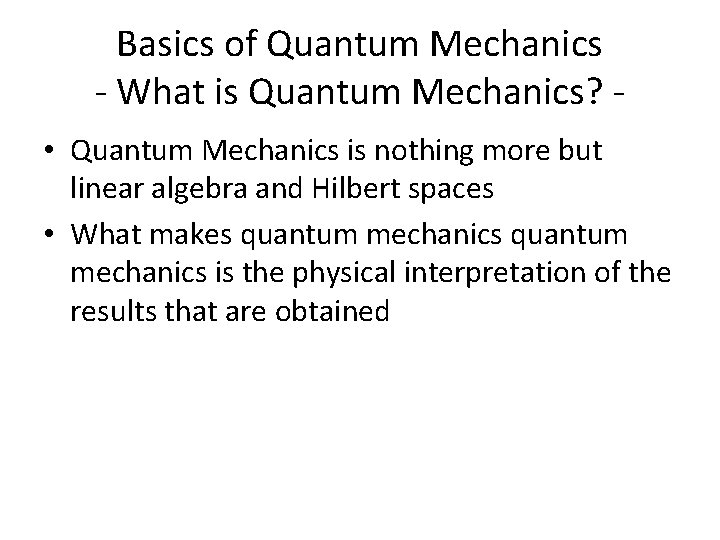Basics of Quantum Mechanics - What is Quantum Mechanics? • Quantum Mechanics is nothing more but linear algebra and Hilbert spaces • What makes quantum mechanics is the physical interpretation of the results that are obtainedBasics of Quantum Mechanics - First Postulate of Quantum Mechanics Quantum physicists are interested in all kinds of physical systems (photons, conduction electrons in metals and semiconductors, atoms, etc. ). State of these rather diverse systems are represented by the same type of functions STATE FUNCTIONS. First postulate of Quantum mechanics: Every physically-realizable state of the system is described in quantum mechanics by a state function that contains all accessible physical information about the system in that state. – Physically realizable states that can be studied in laboratory – Accesible information the information we can extract from the wavefunction – State function of position, momentum, energy that is spatially localized.Basics of Quantum Mechanics - First Postulate of Quantum Mechanics If and represent two physically-realizable states of the system, then 1 2 the linear combination where c 1 and c 2 are arbitrary complex constants, represents a third physically realizable state of the system. Note: Wavefunction (x, t) position and time probability amplitude Quantum mechanics describes the outcome of an ensemble of measurements, where an ensemble of measurements consists of a very large number of identical experiments performed on identical noninteracting systems, all of which have been identically prepared so as to be in the same state.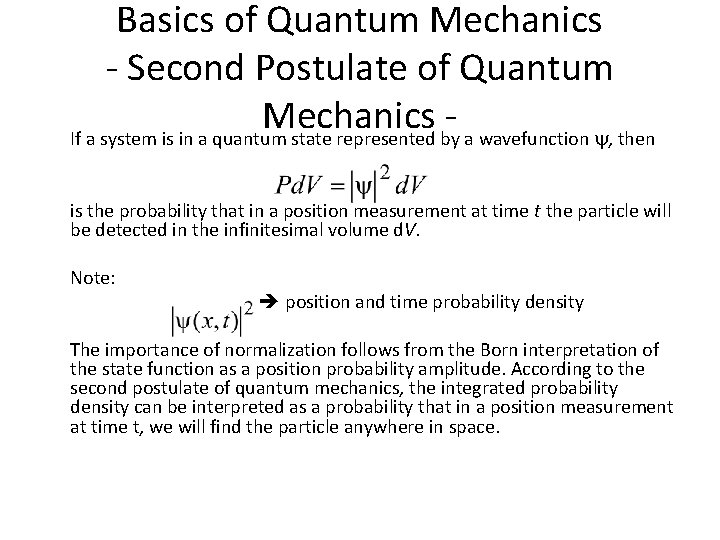Basics of Quantum Mechanics - Second Postulate of Quantum Mechanics If a system is in a quantum state represented by a wavefunction , then is the probability that in a position measurement at time t the particle will be detected in the infinitesimal volume d. V. Note: position and time probability density The importance of normalization follows from the Born interpretation of the state function as a position probability amplitude. According to the second postulate of quantum mechanics, the integrated probability density can be interpreted as a probability that in a position measurement at time t, we will find the particle anywhere in space.Basics of Quantum Mechanics - Second Postulate of Quantum Mechanics - Therefore, the normalization condition for the wavefunction is: Limitations on the wavefunction: – Only normalizable functions can represent a quantum state and these are called physically admissible functions. – State function must be continuous and single valued function. – State function must be a smoothly-varying function (continuous derivative).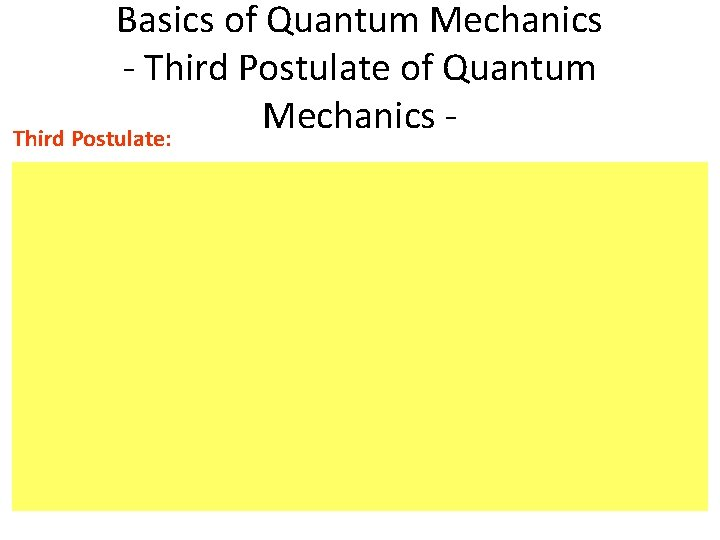Basics of Quantum Mechanics - Third Postulate of Quantum Mechanics Third Postulate:Basics of Quantum Mechanics - More on Operators -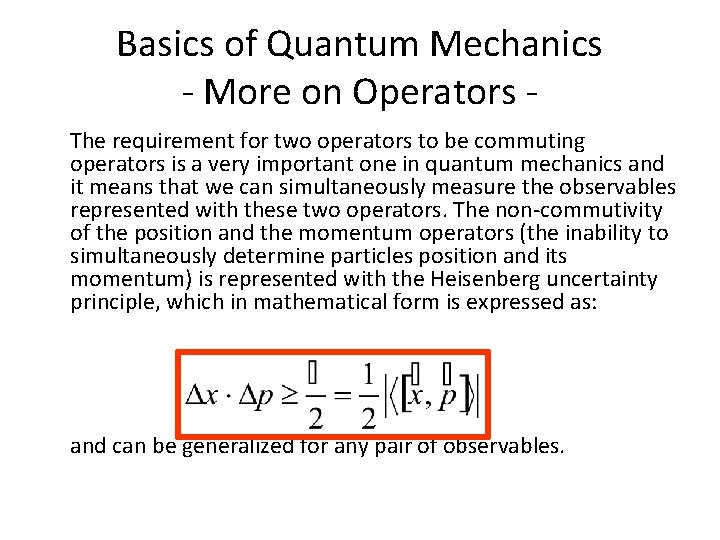Basics of Quantum Mechanics - More on Operators The requirement for two operators to be commuting operators is a very important one in quantum mechanics and it means that we can simultaneously measure the observables represented with these two operators. The non-commutivity of the position and the momentum operators (the inability to simultaneously determine particles position and its momentum) is represented with the Heisenberg uncertainty principle, which in mathematical form is expressed as: and can be generalized for any pair of observables.Basics of Quantum Mechanics - Fourth Postulate of Quantum Mechanics -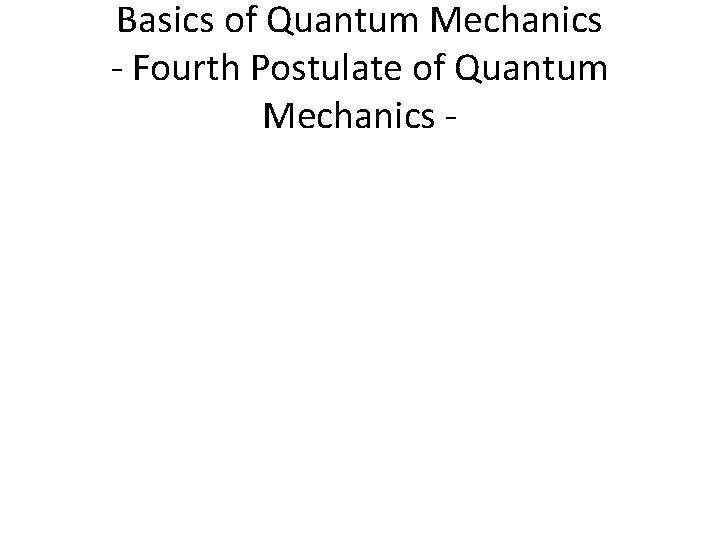Basics of Quantum Mechanics - Fourth Postulate of Quantum Mechanics -